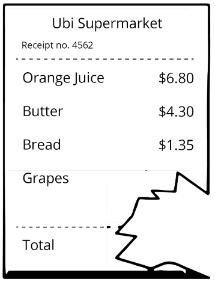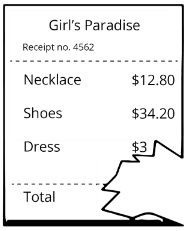Study P3 Mathematics Money - Geniebook# Money

1. Finding the amount of money at first
2. Finding the amount of money left
3. Finding the cost of item

## 1. Finding The Amount Of Money At First

Question 1:

A bag cost $$35$$. After buying the bag, Melissa had $$15$$ left. How much money did she have at first?

Solution:$$35 + 15 = 50$$

She had $$50$$ at first.

$$50$$

Question 2:

The cost of a hairdryer is $$44.95$$. After buying the hairdryer, Mrs Kumar had $$16.40$$ left. How much money did she have at first?

Solution:

To find the amount of money Mrs Kumar had at first, we need to add the cost of the hairdryer and the amount of money left. Remember to align the decimals.$$44.95 + 16.40 = 61.35$$

She had $$61.35$$ at first.

$$61.35$$

Question 3:

After buying $$2$$ basketballs that costs $$28.80$$ each, Michael had $$7.40$$ left. How much money did he have at first?

Solution:\begin{align} 28.80 2 &= 57.60\\[2ex] 57.60 + 7.40 &= 65.00 \end{align}

He had $$65$$ at first.

$$65$$

Question 4:

After paying $$98$$ for a bird and $$25.50$$ on a bird cage, Mr Lim had $$49$$ left. How much money did he have at first?

Solution:\begin{align} 98.00 + 25.50 &= 123.50\\[2ex] 123.50 + 49.00 &= 172.50 \end{align}

He had $$172.50$$ at first.

$$172.50$$

Question 5:

Joyce bought a doll and a board game. The board game cost $$19.70$$. The doll cost $$22.50$$ more than the board game. She gave the cashier some money and received $$4.30$$ change. How much did she give the cashier?

Solution:Cost of the doll\begin{align}\\ &= 19.70 + 22.50\\ &= 42.20 \end{align}
Total cost of the doll and the board game\begin{align}\\ &= 19.70 + 42.20\\ &= 61.90 \end{align}
Amount of money she gave the cashier\begin{align}\\ &= 61.90 + 4.30\\ &= 66.20 \end{align}

She gave the cashier $$66.20$$.

$$66.20$$

Question 6:

Ryan bought a pair of shoes for $$79.80$$ and a set of toiletries which cost $$63.30$$ less than the pair of shoes. After paying the cashier, he received $$3.70$$ change. How much did he give the cashier?

Solution:Cost of the toiletries\begin{align}\\ &= 79.80 - 63.30\\ &= 16.50 \end{align}
Total cost of the shoes and the toiletries\begin{align}\\ &= 79.80 + 16.50\\ &= 96.30 \end{align}
Amount of money he gave the cashier\begin{align}\\ &= 96.30 + 3.70\\ &= 100.00 \end{align}

He gave the cashier $$100$$.

$$100$$

## 2. Finding The Amount Of Money Left/Change Received

Question 1:

Melissa had $$50$$. She bought a bag for $$30$$. How much money did she have left?

Solution:$$50 - 30 = 20$$

She had $$20$$ left.

$$20$$

Question 2:

Mr Tan bought a television set for $$699$$. He gave the cashier $$1000$$. How much change should he receive?

Solution:$$1000 - 699 = 301$$

He should receive $$301$$ change.

$$301$$

Question 3:

Victor bought $$2$$ watches that cost $$101.95$$ each. He paid the cashier $$250$$. How much change should he receive?

Solution:Cost of 2 watches\begin{align}\\ &= 101.95 \times 2\\ &= 203.90 \end{align}
Change\begin{align}\\ &= 250.00 - 203.90\\ &= 46.10 \end{align}

He should receive $$46.10$$ change.

$$46.10$$

Question 4:

Zoe bought a pair of shoes for $$95.80$$ and a pair of socks for $$4.90$$. She paid the cashier $$110$$. How much change should she receive?

Solution:Total cost of the shoes and the socks\begin{align}\\ &= 95.80 + 4.90\\ &= 100.70 \end{align}
Change\begin{align}\\ &= 110.00 - 100.70\\ &= 9.30 \end{align}

She should receive $$9.30$$ change.

$$9.30$$

Question 5:

Jeremy had $$300$$. He paid $$250$$ for his son’s tuition fees and $$28.50$$ for material fees. How much money did he have left?

Solution:Amount of money spent\begin{align}\\ &= 250.00 + 28.50\\ &= 278.50 \end{align}
Amount of money left\begin{align}\\ &= 300.00 - 278.50\\ &= 21.50 \end{align}

He had $$21.50$$ left.

$$21.50$$

## 3. Finding The Cost Of Item

Question 1:

Melissa bought a bag. She gave the cashier $$100$$ and received a $$40$$ change. How much did the bag cost?

Solution:$$100 - 40 = 60$$

The bag cost $$60$$.

$$60$$

Question 2:

Tom bought a T-shirt at a shop. He paid the cashier $$40$$ and received a change of $$16.25$$. How much did the T-shirt cost?

Solution:$$40.00 - 16.25 = 23.75$$

The T-shirt cost $$23.75$$.

$$23.75$$

Question 3:

Mark bought a notebook at a shop. He paid the cashier $$50$$ and received a change of $$18.45$$. How much did the notebook cost?

Solution:$$50.00 - 18.45 = 31.55$$

The notebook cost $$31.55$$.

$$31.55$$

Question 4:

Mrs Chan went to Ubi supermarket and bought some items. She paid the cashier $$50$$ and received a change of $$34.35$$. However, she accidentally tore the receipt as shown. How much did the grapes cost?Solution:

Amount of money spent\begin{align}\\ &= 50 - 34.35\\ &= 15.65 \end{align}
Total cost of the orange juice, the butter and the bread\begin{align}\\ &= 6.80 + 4.30 + 1.35\\ &= 12.45 \end{align}
Cost of the  grapes\begin{align}\\ &= 15.65 - 12.45\\ &= 3.20 \end{align}

The grapes cost $$3.20$$.

$$3.20$$

Question 5:

Kim went shopping and bought some items. She paid the cashier $$100$$ and received a change of $$13.50$$. She accidentally tore the receipt as shown. How much did the dress cost?Solution:

Amount spent\begin{align}\\ &= 100 - 13.50\\ &= 86.50 \end{align}
Total cost of the necklace and the shoes\begin{align}\\ &= 12.80 + 34.20\\ &= 47.00 \end{align}
Cost of the dress\begin{align}\\ &= 86.50 - 47.00\\ &= 39.50 \end{align}

The dress cost $$39.50$$.

$$39.50$$

Continue Learning
Volume Money
Mass Fractions
Addition And Subtraction Whole Numbers 1
Whole Numbers 3 Whole Numbers 6
Bar Graphs Angles
Multiplication and Division Length, Mass And Volume
Length Area and Perimeter
Parallel And Perpendicular LinesPrimaryPrimary 1Primary 2Primary 3EnglishMaths
Volume
Money
Mass
Fractions
Whole Numbers 1
Whole Numbers 3
Whole Numbers 6
Bar Graphs
Angles
Multiplication and Division
Length, Mass And Volume
Length
Area and Perimeter
Parallel And Perpendicular LinesSciencePrimary 4Primary 5Primary 6SecondarySecondary 1Secondary 2Secondary 3Secondary 4